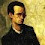Ir al contenido principal

## Due February 21st

### Problem 1

1. Verify explicitly that $\text{Ind}(\partial D(z_0,r),z_0) = 1$ for all $z_0\in\mathbb C$ and $r>0$.
2. For $z_0\in\mathbb C$ and $r>0$,
$\text{Ind}(\partial D(z_0,r),z) = \begin{cases} 1 & |z-z_0| < r\\ 0 & |z-z_0| > r. \end{cases}$

### Problem 2

1. If $f,g$ are holomorphic near $z_0$ and $f$ has a simple zero at $z_0$, find an expression for the residue of $g/f$ at $z_0$.
2. If $f$ has a simple pole at $z_0$ and $g$ is holomorphic near $z_0$, then
$\text{Res}(fg,z_0) = g(z_0)\text{Res}(f,z_0)$.
3. If $f$ is holomorphic near $z_0$ and $g(z) = f(z)/(z-z_0)^n$, then
$\text{Res}(g,z_0) = \dfrac{f^{(n-1)}(z_0)}{(n-1)!}$.

### Problem 3

1. Use the residue theorem to show that $\displaystyle \int_{-\infty}^\infty \frac{dx}{1+x^2} = \pi$. (Hint: For $R>0$, consider the upper semicircle $\gamma$ with radius $R$ and diameter over the real line, calculate $\int_\gamma f(z)dz$ and let $R\to\infty$.)
2. Let $P,Q$ polynomials over $\mathbb R$, $\text{degree}(Q)\ge\text{degree}(P)+2$, and suppose $Q$ does not have real roots. Then $\displaystyle \int_{-\infty}^\infty \frac{P(x)}{Q(x)} dx$ is $2\pi i$ times the sum of the residues of $P(z)/Q(z)$ in the upper half-plane.

### Problem 4

1. Find $\displaystyle \lim_{\epsilon\to0^+,R\to\infty} \Big( \int_{-R}^{-\epsilon} + \int_\epsilon^R\Big) \frac{e^{it}}{t} dt$. (Hint: Consider the same curve $\gamma$ as in the previous exercise, with a small dent of radius $\epsilon$ around $0$.)
2. Use the previous result to calculate the integral $\displaystyle \lim_{R\to\infty} \int_{-R}^R \frac{\sin t}{t} dt$.

### Problem 5

Suppose $P(z)$ is polynomial over $\mathbb C$ of degree $n\ge2$ with distinct roots $z_1, z_2, \ldots, z_n$. Then
$\displaystyle \sum_{j=1}^n \frac{1}{P'(z_j)} = 0$.
(Explain first why each $P'(z_j)\not=0$.)

### Comentarios

1.En el problema 2.1 tenemos que asumir que g(Zo) no es cero?

1.No, g(z0) puede ser cero.

2.Gracias. En el problema 4.2 es tomando el límite?

3.Sí, ya está corregido.